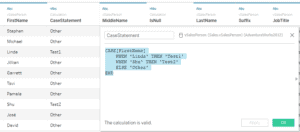# How do I write a Case Statement With Multiple Conditions in Tableau?

• Tableau FAQs
• December 13, 2018

I have two conditions using separate fields, but Tableau won’t let me write it as I would like in SQL? CASE ([Expression1]) WHEN 2 AND WHEN ([Expression1]) ‘XXXX’ THEN 1 ELSE 0 END)

In some cases, an IF expression cannot be used, and then you can use a CASE expression. You can also use CASE with an IF statement, but we’ll look at the simplest way:

``````CASE[FirstName]

WHEN 'Linda' THEN 'Test1'

WHEN 'Shu' THEN 'Test2'

ELSE 'Other'

END``````

CASE[FirstName] – first, you need to specify the expression with which you will perform calculations

WHEN ‘Linda’ THEN ‘Test1’ – then we need to specify what value in the field you need to find to show the result

WHEN ‘Shu’ THEN ‘Test2’ – you can use multiple values to show the result of an expression

ELSE ‘Other’ – at the end, you need to specify the result if the previous expression is FALSE

END – and here we end the function## Related Posts

### How do I calculate ratios using the data in two columns t...

• Tableau FAQs
• December 13, 2018

### In Tableau, how do I use DATEDIFF to calculate number of ...

• Tableau FAQs
• December 13, 2018

### Is there a way to merge or append Excel data files into o...

• Tableau FAQs
• December 13, 2018